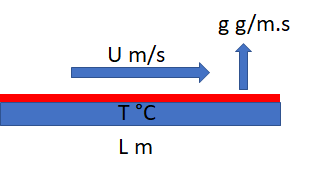## Solvent Evaporation

### Quick Start

Solvent evaporation depends on parameters specific to each solvent, plus air temperature and velocity. A simple model gives an evaporation time for a chosen thickness of solvent, a more academic model lets you see how the quick model works.

### Solvent Evaporation

Solvent
Airflow m/s
T °C
Thickness μm
tEvap

Note that up to 5 March 2021 there was an error of dividing, rather than multiplying, by density so that evaporation times were too slow by a factor of ρ² which was OK for water but not for other solvents. I thank the user who found the bug.

Now the more academic app which shows in more detail what is going on. The calculation method is the same, but for simplicity the quick model assumes that L=1000.

### Flat Plate Evaporation

Airflow m/s
T °C
Length mm
Antoine A
Antoine B
Antoine C
VP mm/Hg
D cm²/s
ν cm²/s
Re
Sc
Sh
k m/s
mole/m.s
g/m.sWe often need to know the evaporation rate of a solvent on a flat plate with a steady air flow above it. This is rather difficult to calculate from first principles, but some well-known approximations are good enough given the uncertainties in, say, measuring the rate.

The calculation uses a set of dimensionless numbers. Based on the air velocity, U, the temperature T and length of the plate L, then basic parameters such as the kinematic viscosity of air, ν, diffusion coefficient of the solvent in air, D (itself a complicated dependency on MWt and MVol of the solvent) we have:

 Reynolds Number Re UL/ν Schmidt Number Sc ν/D Sherwood Number Sh kL/D

The key number is Sh because it contains the mass transfer coefficient, k. It is calculated from the other two via:

Sh = 0.664Re^0.5Sc^0.333

So we get k from:

k=(ShD)/L

From k we can calculate the moles of solvent evaporated per unit width along the length L, given a molar concentration c at the surface and assuming that the air is solvent-free:

Evap = k.L.c

The molar concentration c depends on the vapour pressure, VP, the temperature T and the universal gas constant R:

c = "VP"/(RT)

The mass evaporated is simply the molar rate multiplied by the moelcular weight, Evap*MWt. Finally, we can calculate the vapour pressure if we know the Antoine Constants of the solvent:

log_10(VP) = "AA"-"AB"/(AC+T)

There are many different units used in Antoine. Here pressure is in mm Hg and T is in °C. Why? Because the most common Antoine databases use them.

A few details remain. The air properties assume dry air - the calculated evaporation rates for moist air are not significantly different at this level of approximation. The kinematic viscosity of air changes with temperature; a simple polynomial is used to calculate it. The diffusion coefficient of the solvent in air can be calculated from T, MWt and the molar volume, MVol via a formula not shown here. The solvent values of MWt, MVol, AA, AB, AC are extracted from the values shown in the combobox for each solvent.